← Back to Community
##### Simple Depth First Search
Profile icon
[deleted]

Depth first search starts at the root node and goes as far as it can down the a given path until it reaches a leaf node, then it backtracks until it finds an unexplored path and explore it. This process it repeated until all nodes in the graph are explored.
Let’s see how depth first search works with an example.

#### 1)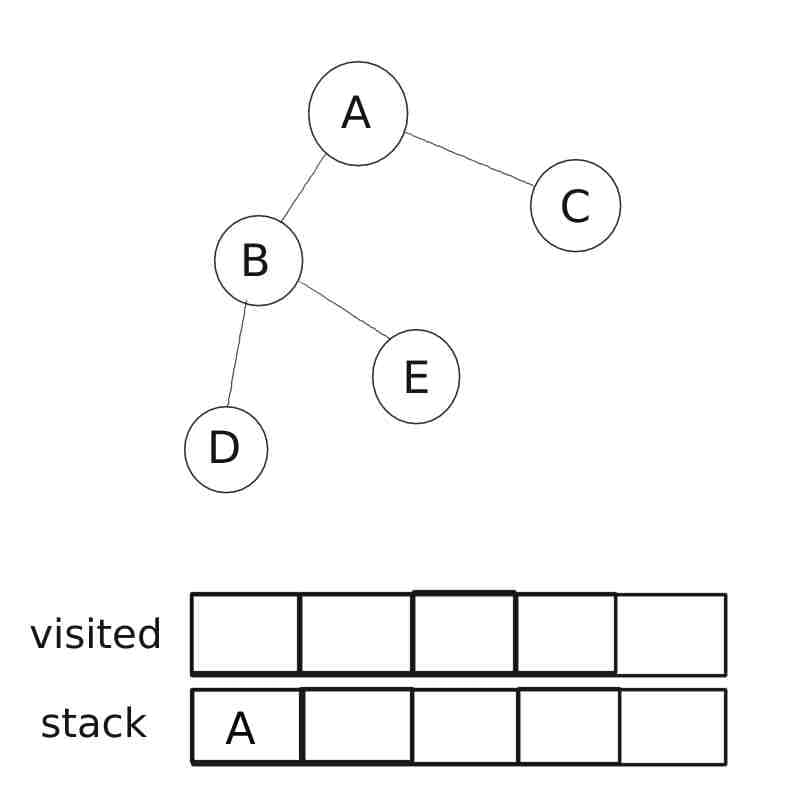We start from node 'A' and add it to the stack.

#### 2)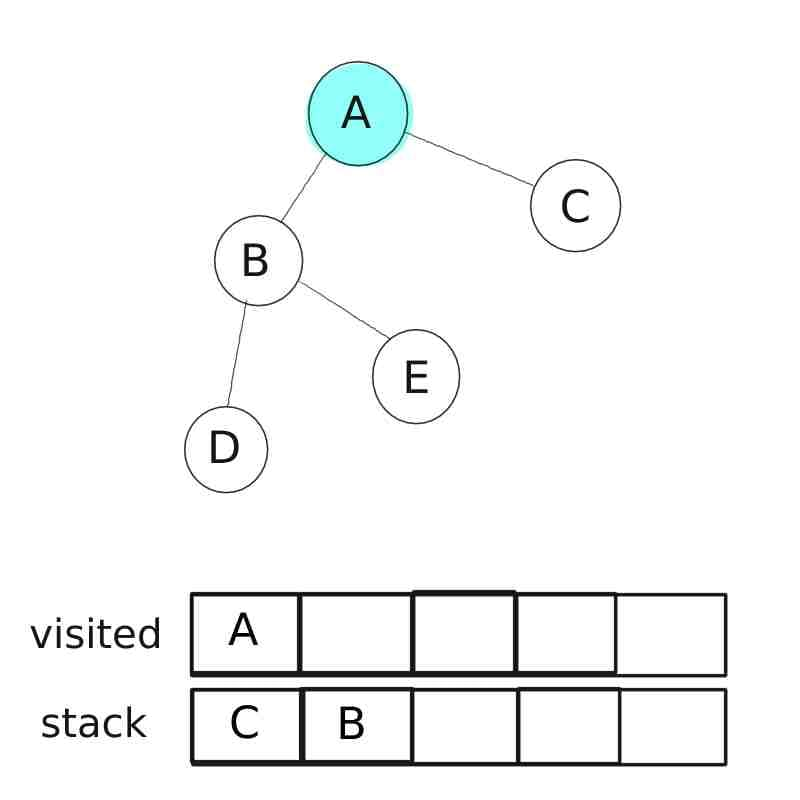We pop node 'A' out of the stack and add to the visited list. Then we put all of its neighbor in the stack, namely node 'B' and node 'C'

#### 3)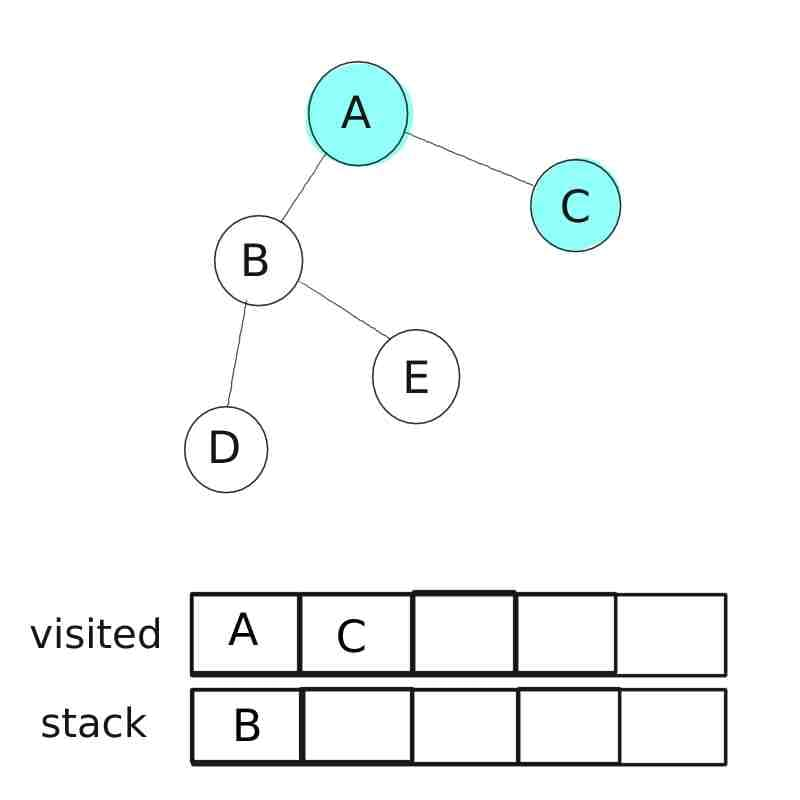We pop node 'C' out of stack and put it in visited list. Since all neighbor of node 'C' are visited we move to the node 'B'.

#### 4)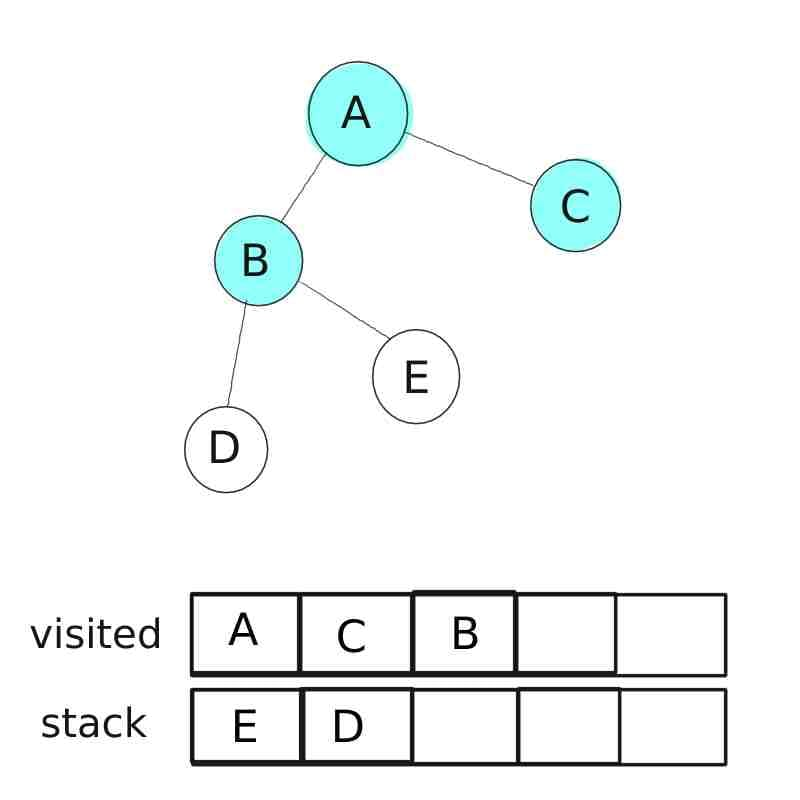We pop node 'C' out of stack and put it in visited list. Since node 'A' is already visited we put remaining neighbors i.e. node 'D' and node 'E' into the stack.

#### 5)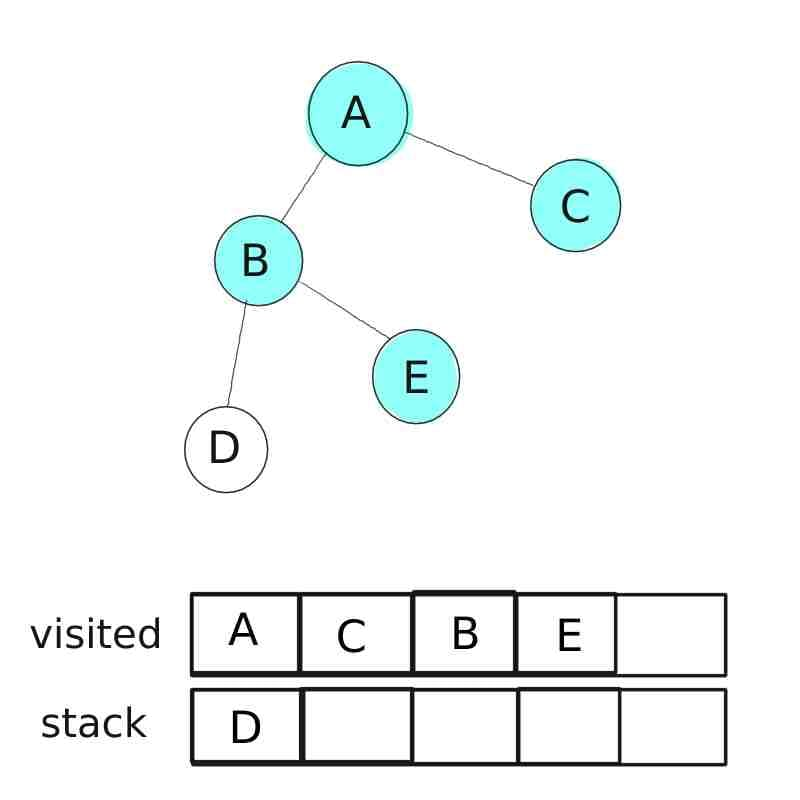We pop node 'E' out of stack and put it in visited list. Since all neighbor of node 'E' are visited we move to the node 'D'.

#### 6)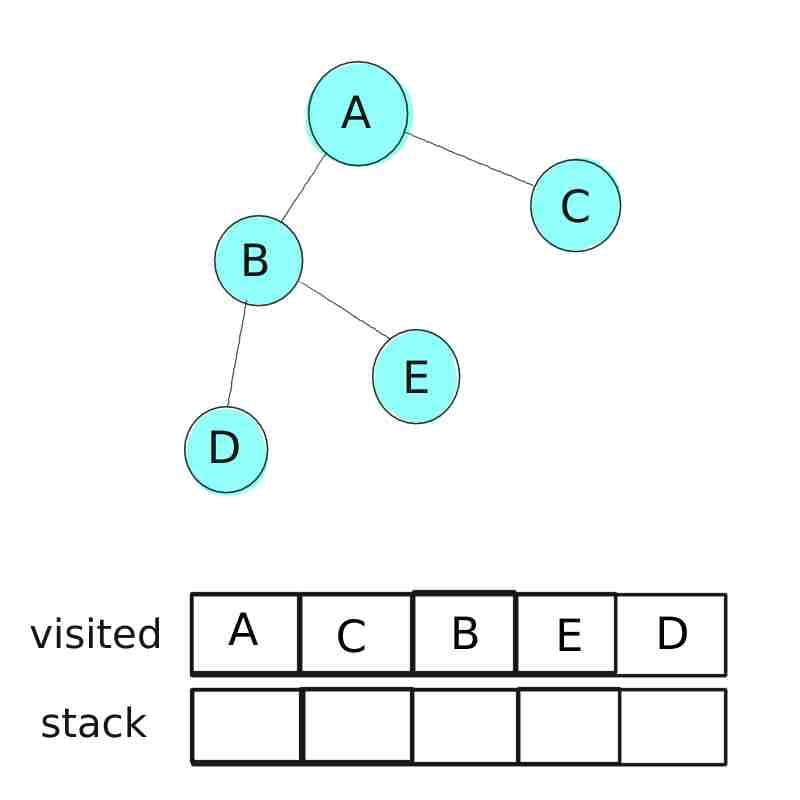We pop node 'D' out of stack and put it in visited list. Since all neighbor of node 'D' are visited, noting is added to stack.

Now all of the nodes in the given graph are visited, so we have done traversing given graph using depth first search.

### Depth first search graph traversing using python.css-1q3m8ps{margin-left:var(--space-4);margin-right:var(--space-4);display:none;}.css-1gcl232{min-width:16px;min-height:16px;}

We are going to use same graph we use in example above.

```.css-19sk4h4{position:relative;}.css-1bu6gr6{-webkit-align-items:stretch;-webkit-box-align:stretch;-ms-flex-align:stretch;align-items:stretch;border-width:0;border-style:solid;box-sizing:border-box;display:-webkit-box;display:-webkit-flex;display:-ms-flexbox;display:flex;-webkit-flex-basis:auto;-ms-flex-preferred-size:auto;flex-basis:auto;-webkit-flex-direction:column;-ms-flex-direction:column;flex-direction:column;-webkit-flex-shrink:0;-ms-flex-negative:0;flex-shrink:0;outline:none;min-height:0;min-width:0;position:relative;}.css-1n2m10r{padding:var(--space-8);border-radius:var(--border-radius-4);background-color:var(--background-higher);}.css-1hwur6u{-webkit-align-items:stretch;-webkit-box-align:stretch;-ms-flex-align:stretch;align-items:stretch;border-width:0;border-style:solid;box-sizing:border-box;display:-webkit-box;display:-webkit-flex;display:-ms-flexbox;display:flex;-webkit-flex-basis:auto;-ms-flex-preferred-size:auto;flex-basis:auto;-webkit-flex-direction:column;-ms-flex-direction:column;flex-direction:column;-webkit-flex-shrink:0;-ms-flex-negative:0;flex-shrink:0;outline:none;min-height:0;min-width:0;padding:var(--space-8);border-radius:var(--border-radius-4);background-color:var(--background-higher);}.css-1svvr0w{height:0;}.css-rk73ff{padding:var(--space-4);padding-left:var(--space-4);padding-right:var(--space-2);font-family:var(--font-family-code);font-size:14px;line-height:var(--line-height-small);overflow-x:auto;tab-size:2;word-break:break-word;white-space:break-spaces;overflow-wrap:anywhere;}```def dfs(graph, start):
#initializing empty stack and visited list
stack=[]
visited = []

stack.append(start)

#loop will execute as long as stack is not empty
while(stack):

#pop top element from the stack
N = stack.pop(-1)

#if node not in visited list then add it to visited list
if N not in visited:
visited.append(N)

#this allow us to detect the leaf nodes and prevent algorithm from further exploring it
if N not in graph:
continue

#loop through all node connected to root node
for node in graph[N]:
if node not in visited:
stack.append(node)

#return traversed nodes list
return visited

# undirected graph
graph = {
'A' : ['B' , 'C'],
'B' : ['D' , 'E'],
'C' : ['A'],
'D' : ['B'],
'E' : ['B']
}

# printing output
print ( dfs(graph, 'A') )``````
``````# output

['A', 'C', 'B', 'E', 'D']``````

### Using depth first search to find the shortest find between node 'A' and 'F' in given graph.

This file cannot be displayed: images/depth_first_search/depth_first_graph.jpg

Here we are going to recursive approach to find the path, each time the function call itself with the generated path and current node. If path exist then it return it.

``````
def find_path(graph, start, end, path = []) :
#if start node not in path then return none
if start not in graph:
return None

#initialize the path with starting node
path = path + [start]

#if if find end node then return path
if start == end:
return path

#loop over all of its neighbor
for node in graph[start]:
if node not in path:

#pass the generated path and current node
new_path = find_path(graph, node, end, path)

#if new_path exist return it
if new_path:
return new_path

#if path does not exit return None
return None

if __name__=='__main__':

#graph implementation using dictionary
graph = {
'A':['B', 'C', 'D'],
'B':['A' , 'D', 'E'],
'C':['A', 'D'],
'D':['B', 'A', 'C'],
'E':['B', 'F'],
'F':['E']
}

#print the path in terminal

print ( find_path(graph, 'A' , 'F'))``````
``````
#output

['A', 'B', 'E', 'F']``````
1. Finding path between two given nodes.
2. Detecting cycles in the graph.
1. Depth first search does not take path weight into a consideration, it means it does not guarantee shortest path.

2. Time complexity of the depth first search algorithm is O (V+E). Here V is number of nodes and E is number of edges.

### Reference

Python Patterns - Implementing Graphs

Voters
Profile icon
DynamicSquid